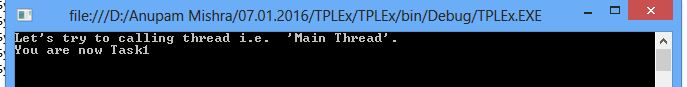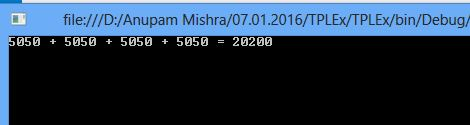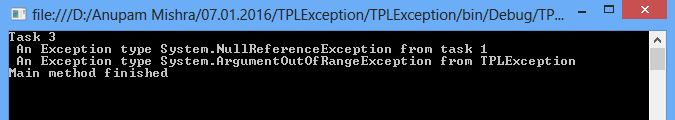In my previous article, we have learned about Parallel Programming in C# .Now we pick after this and discuss about Task Parallel Library (TPL). The TPL is an important improvement over the previous models such as Asynchronous Programming Model (APM), Event-Based Asynchronous Pattern (EAP) etc. It is a way better simplifies of parallel processing and makes good use of system resources. If you want to use parallel processing in your programs, TPL is the convenient solution.

The Task Parallel Library (TPL) is a basic concept of a task (i.e. a unit of work or operation), which represents an asynchronous operation. The term “Task parallelism” refers to one or more independent asynchronous tasks running concurrently i.e. in parallel.

TPL is the preferred API for writing multi-threaded, asynchronous and parallel code applications. The Task Parallel Library (TPL) supports data parallelism through the System.Threading.Tasks.Parallel class. This class provides method-based parallel implementations of for and foreach loops. We can write the loop logic for a Parallel.For or Parallel.ForEach loop same as we would write a sequential loop. You do not have to create threads or queue work items. In basic loops, you do not have to use locks.

Here, we can create tasks implicitly or explicitly .To create a task implicitly, we can use

The Parallel Class— a static class that has three methods: For, ForEach and Invoke. For and ForEach  allow loops to run task in parallel whereas Invoke() method executes each of the provided tasks/actions, possibly in parallel.

The Parallel. Invoke () method provides a convenient way to run any number of arbitrary statements concurrently. Just pass in an Action delegate for each item of work. The easiest way to create these delegates is to use lambda expressions. The lambda expression can either call a named method or provide the code inline. Here, we use inline code for lambda expression.

Parallel.Invoke(() => DoSomeWork(), () => DoSomeOtherWork());

You can use the TaskFactory.StartNew () method to create and start a task in one operation. To create and start a task in one operation as shown in the following example.

`using System;using System.Threading;using System.Threading.Tasks; public class PLINQEx{    public static void Main()    {        Thread.CurrentThread.Name = 'Main Thread';        //  Create and start the task in one operation.         Task task1 = Task.Factory.StartNew(() => Console.WriteLine('You are now Task1'));         // Output a message from the calling thread.       Console.WriteLine(@'Let's try to calling thread i.e.  '{0}'.', Thread.CurrentThread.Name);        task1.Wait();        Console.ReadKey();    }} `

##### Output:`using System;using System.Threading;using System.Threading.Tasks;public class TaskEx{    public static void Main()    {        Task<Double>[] taskArray = { Task<Double>.Factory.StartNew(() => Compute()),                                     Task<Double>.Factory.StartNew(() => Compute()),                                      Task<Double>.Factory.StartNew(() => Compute()),                                      Task<Double>.Factory.StartNew(() => Compute())};        var result = new Double[taskArray.Length];        Double sum = 0;         for (int i = 0; i < taskArray.Length; i++)        {            result[i] = taskArray[i].Result;            Console.Write('{0} {1}', result[i],                              i == taskArray.Length - 1 ? '= ' : '+ ');            sum += result[i];        }        Console.WriteLine('{0}', sum);        Console.ReadKey();    }    private static Double Compute()    {        Double sum = 0;        for (var value = 0; value <= 100; value ++)            sum += value;        return sum;    }}`
##### Output:##### Waiting for tasks to finish:

When the final results is computed by a task, the main thread waits until the task is not finished. In this case, we will wait for some time to get the computed results. Also this will help us with handling the exceptions that might be thrown from the task.

If we are not defining exception code in our task, the application will might crash when an exception is thrown, leaving an old user experience behind. In parallel programming, it is even more important since the application could behave unpredictably. TPL has provided a consistent model for handling exception in task execution.

When an exception occurs within a task (and is not caught), it is not thrown immediately. Instead, it is squirreled away by the .Net framework and thrown when some trigger member method is called, such as Task.Result, Task.Wait(), Task.WaitAll() or Task.WaitAny(). In this case, an instance of System.AggregateException is thrown that acts as a wrapper around one or more exceptions that have occurred. This is important for methods that coordinate multiple tasks like Task.WaitAll() and Task.WaitAny(), so the AggregateException is able to wrap all the exceptions within the running tasks that have occurred.

In the following example, we are creating three tasks and start them immediately, in these three task, two task can throw any type  of exception. The main calling thread calls the WaitAll() method and catches the AggregateException. Finally, it iterates through the InnerExceptions property and prints out the details regarding the thrown exceptions. This property is the wrapper holding all the information about the aggregated exceptions.

`using System;using System.Threading.Tasks;namespace TPLException{    class Program    {        static void Main(string[] args)        {            Task task1 = new Task(() =>            {                NullReferenceException exception = new NullReferenceException();                exception.Source = 'task 1 ';                throw exception;            });            Task task2 = new Task(() =>            {                throw new ArgumentOutOfRangeException();            });             Task task3 = new Task(() =>            {                Console.WriteLine('Task 3');            });            task1.Start();            task2.Start();            task3.Start();            try            {                Task.WaitAll(task1, task2, task3);            }            catch (AggregateException ex)            {                foreach (Exception inner in ex.InnerExceptions)                {            Console.WriteLine(@' An Exception type {0} from {1}',                                                 inner.GetType(), inner.Source);                }            }            Console.WriteLine(@'Main method finished');            Console.ReadKey();        }    }}`
##### Output: